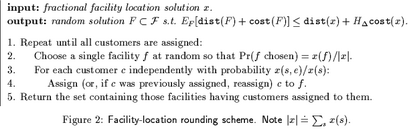## neal young / Young00Kmedians

•The paper gives approximation algorithms for the $$k$$-medians and facility-location problems (both NP-hard). For $$k$$-medians, the algorithm returns a solution using at most $$\ln(n+n/\epsilon) k$$ medians and having cost at most $$(1+\epsilon)$$ times the cost of the best solution that uses at most $$k$$ medians. Here $$\epsilon>0$$ is an input to the algorithm. In comparison, the best previous algorithm [Lin and Jeff Vitter, 1992] had a $$(1+1/\epsilon)\ln n$$ term instead of the $$\ln(n+n/\epsilon)$$ term in the performance guarantee. For facility location, the algorithm returns a solution of cost at most $$d+\ln(n) k$$, provided there exists a solution of cost $$d+k$$ where $$d$$ is the assignment cost and $$k$$ is the facility cost. In comparison, the best previous algorithm [Dorit Hochbaum, 1982] returned a solution of cost at most $$\ln(n)(d+k)$$. For both problems, the algorithms currently provide the best performance guarantee known for the general (non-metric) problems.

The paper also introduces a new probabilistic bound (the so-called Chernoff-Wald bound) for bounding the expectation of the maximum of a collection of sums of random variables, when each sum contains a random number of terms. The bound is used to analyze the randomized rounding scheme that underlies the algorithms.